# MatterJS Body Size

## Recommended Posts

Hello, I've recently started to switch to using matterjs for physics rather than the built in arcade physics for my game as I have determined the latter is unsuitable for what I'm doing. However I'm having a hard time understanding how setting the size for a sprite body is supposed to work. I'll try to show what I mean. I have a player sprite, the dimensions for it's frame is 24x32.

So without doing anything other than adding it as a matter sprite, this (the screenshot below) is the default body boundary as shown by matter's debug graphic, it's a rectangle which is the same size as the sprite (24x32).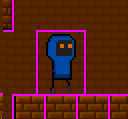Now obviously I don't want it to be like this, I want the body to be just a bit smaller than the sprite. Now when I was in arcade physics I would do something like this:

``````/* where this.collisionBounds is Phaser.Geom.Rectangle(5, 3, 12, 29) for Player class */
var b = this.collisionBounds;
this.body.setSize(b.width, b.height);
this.body.setOffset(b.x, b.y);
``````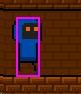For this I get this screenshot, which is perfect when I'm using arcade physics.

However when I try to do the equivalant in matterjs, things get a bit weird for me. I use the following code:

``````var x = this.x;
var y = this.y;
var b = this.collisionBounds; // this.collisionBounds is still Phaser.Geom.Rectangle(5, 3, 12, 29) (same as before)
var spriteBody = Matter.Bodies.rectangle(b.x, b.y, b.width, b.height); // i know i should have a chamfer but I'm just leaving that out for now to make things easier to see
this.physics.setExistingBody(spriteBody);
this.physics.setFixedRotation();
this.physics.setPosition(x, y);``````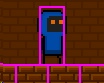and now i get this.

This is the first point where I'm confused, I really can't figure out what is happening here. The body has shrunk slightly (by 1px?) and seems to have moved upwards realtive to the sprite. Now what's really strange is if I change the fifth line in the previous block of code to this:

``var spriteBody = Matter.Bodies.rectangle(0, 0, b.width, b.height)``

So that the x and y offset for the body is always (0, 0) it appears to have no effect at all:wuttt????

Now perhaps if we ignore our problems, they might just go away. Let's continue on to the next step anyway, add some sensors:

``````this.physics = this.level.matter.add.gameObject(this, {});
var b = this.collisionBounds;
var spriteBody = Matter.Bodies.rectangle(0, 0, b.width, b.height);

var sensors = {
bottom: Matter.Bodies.rectangle(0, (b.height * 0.5) + b.y, b.width * 0.5, 2, { isSensor: true}),
left: Matter.Bodies.rectangle((-b.width * 0.5) - 1, (b.height * 0.1) + b.y, 2, b.height * 0.5, { isSensor: true }),
right: Matter.Bodies.rectangle((b.width * 0.5) + 1, (b.height * 0.1) + b.y, 2, b.height * 0.5, { isSensor: true }),
};
const compoundBody = Matter.Body.create({
parts: [spriteBody, sensors.bottom, sensors.left, sensors.right],
friction: 0
});
this.physics.setExistingBody(compoundBody);

this.physics.setFixedRotation();
this.physics.setPosition(x, y);``````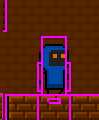Okay same thing as before, but now we have sensors. Another thing I'm confused about is why the sensors are centered about the center of the sprite but the main body isn't? This just makes no sense to me.

So now I try changing this:

``var spriteBody = Matter.Bodies.rectangle(0, 0, b.width, b.height)``

back to:

``var spriteBody = Matter.Bodies.rectangle(b.x, b.y, b.width, b.height)``

now it actually has an effect (albeit an undesired one):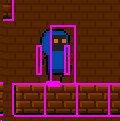Why is the main body now offset now we have added sensors. Why would this make a difference? I'm so confused.

I feel like I've gone down a very long rabbit hole of misunderstanding. Can someone just please tell me how to get what I could easily get before in arcade physics and apply it to matterjs? Thanks very much.